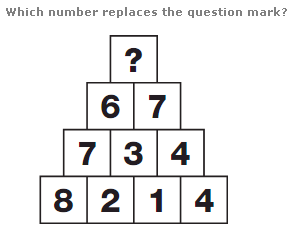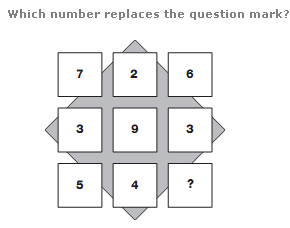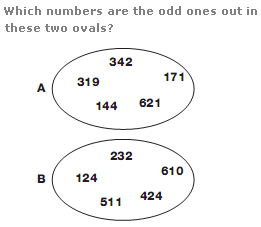# Puzzles - Number puzzles

### Exercise :: Number puzzlesAnswer : 12 Explanation : Working from the bottom row to the apex of the triangle, the sum of the values in each row follows the sequence 15, 14, 13,12.Answer : 6 Explanation : Each row and column of the diagram adds up to 15.Answer : A:319 B:424 Explanation : In the first oval, the sum of the separate digits of each 3 digit number is 9, and in the second oval, the sum of the separate digits is 7.